Polynomials, Factors, and Zeros
 Home > Lessons > Polynomials, Factors, and Zeros Search | Updated September 27th, 2020
Introduction
In this section, you will learn how a polynomial's zeros, factors, and graph are all related. Here are the sections within this lesson:

So that you will be able to understand the lessons that follow, we need to review a few definitions.

 polynomial A polynomial is a mathematical expression of one or more algebraic terms each of which consists of a constant multiplied by one or more variables each raised to nonnegative integral powers, such as…3x2 + 2x - 5. zero A zero is the location where a polynomial intersects the x-axis. These locations are called zeros because the y-values of these locations are always equal to zero. factor A factor is one of the linear expressions of a single-variable polynomial. A polynomial can have several factors, such as the factors...(x - 1) and (x + 3).

When polynomials are graphed, many of them intersect the x-axis. The locations where a polynomial crosses the x-axis are called ‘zeros.’ For instance, we can graph this polynomial.This is the graph of the polynomial.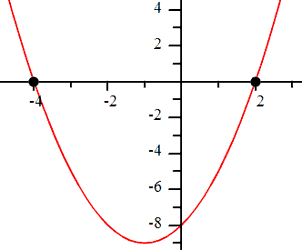Notice how the polynomial intersects the x-axis at two locations. It intersects at (-4, 0) and (2, 0). So, we say the polynomial has zeros at -4 and 2.

Likewise, we can graph this polynomial.This is the graph of the polynomial.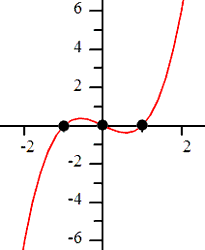It can easily be seen that this polynomial has three zeros, namely -1, 0, and 1.

The factors of a polynomial are important to find because they can be multiplied together to gain a polynomial.     In our lesson on zeros, we saw this graph.We saw that the zeros were -4 and 2. To get the factors, we simply take the opposite of the zeros. These are the factors.We also saw this graph in the last section.It’s zeros are -1, 0, and 1. Here are it’s factors.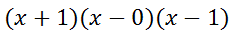The (x – 0) factor is the same thing as writing x. So, here are the factors written more properly.Or, we can write them more compactly as…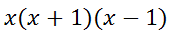uiz: Finding Zeros and Factors, Given a Polynomial

We have an entire section devoted to operations on polynomials, which will come in handy for our next section. You can find it here: Operations on Polynomials. Please review the section before proceeding to the next section.

Besides learning how to add, subtract, multiply and divide polynomials, it is extremely convenient to have a graphing calculator. Modern graphing calculators, like the TI-Nspire CX CAS model, are excellent tools for quickly displaying graphs and consequently speed up the learning process.

We will examine the connection between a polynomial's zeros, factors, and expanded forms.

Let's again revisit this polynomial as our first example.If we look at the graph of this polynomial, we get this picture.It has the zeros at -4 and 2, which means it has the factors (x + 4) and (x - 2).

If we were to multiply the factors (x + 4) and (x - 2), this is what we would get.This simplifies as follows.The above polynomial is the original polynomial. This demonstrates how the graph of a polynomial is related to its zeros and its factors.

For our second example, we should look at the cubic polynomial we saw in a previous section.Viewing this polynomial on a coordinate plane yields this picture below.It has zeros at -1, 0, and 1, which means its factors are...Or, we can write them as...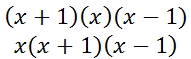If we multiply the (x + 1) and the (x - 1), we get this.This simplifies as follows.Now, we have to multiply this result by the last factor, x.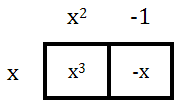This yields our final result and our original polynomial.This indicates how a polynomial's expanded form is related to its zeros and its factors.ideo: Real Zeros to Factors to Polynomialsuiz: Finding a Polynomial, Given its Real Zeros

This video explains how to use complex zeros to gain factors, and a polynomial expression. It also will inform you of complex conjugate pairs.ideo: Complex Zeros to Factors to Polynomialsuiz: Finding a Polynomial, Given its Imaginary Zeros

When graphing polynomials, there are patterns to follow. These activities and quizzes will assist the learning process to graph polynomial equations.ctivity: Translation: Discoveryctivity: Translating Polynomialsuiz: Translations: From Equations to Statementsuiz: Translations: From Statements to Equations

Like there are logical rules that tell us the connections between zeros and factors, there are also rules that govern the end behavior (branches) of a polynomial. Knowing what the y-values are when the x-values are very large and very small will help us sketch the graphs of polynomials.

[Let it be known there is a big difference between even and odd degree and even and odd functions.]

This video will instruct you on the connections between a polynomial's degree, leading coefficient, and its end behavior.ideo: Even and Odd Degree

When we deal with polynomials, two terms emerge: root and zero. There is a distinction between the two even though some people use them interchangeably. Here are their differences:

 root It is the solution of an equation. 2 and -2 are roots ofx2 - 4 = 0 because 2 and -2 are solutions to the equation. zero It is a value for which a function is equal to zero. 5 is a zero forf(x) = x - 5 becausef(5) = 0.

The Rational Root Theorem is an involved concept. Understanding it and using requires several layers of explanation, which is done in this video.ideo: The Rational Root Theoremuiz: Finding the Zeros of a Cubic Polynomialuiz: Finding the (Complex) Zeros of a Cubic Polynomialuiz: Finding the Zeros of a Quartic Polynomial

Try our instructional videos that detail the lessons above.ideo: Polynomials: Factors & Zerosideo: Real Zeros to Factors to Polynomialsideo: Complex Zeros to Factors to Polynomialsideo: Multiplying Polynomialsideo: Dividing Polynomials: Synthetic Divisionideo: Even and Odd Degreeideo: The Rational Root Theorem

Try our interactive quizzes to determine if you understand the lessons above.uiz: Finding Zeros and Factors, Given a Polynomialuiz: Finding a Polynomial, Given its Real Zerosuiz: Finding a Polynomial, Given its Imaginary Zerosuiz: Finding the Zeros of a Cubic Polynomialuiz: Finding the (Complex) Zeros of a Cubic Polynomialuiz: Finding the Zeros of a Quartic Polynomialuiz: Translations: From Equations to Statementsuiz: Translations: From Statements to Equations

Use these activities to learn about polynomials, factors, and zeros.ctivity: Factors and Zerosctivity: Translation: Discoveryctivity: Translation: Discoveryctivity: Translating Polynomials

Try these lessons, which are closely related to the lessons above.esson: End Behavioresson: Operations on Polynomialsesson: Factoring Trinomialsesson: Polynomial Modelsesson: PARCC Problems and Solutionsesson: The Rational Root Theorem